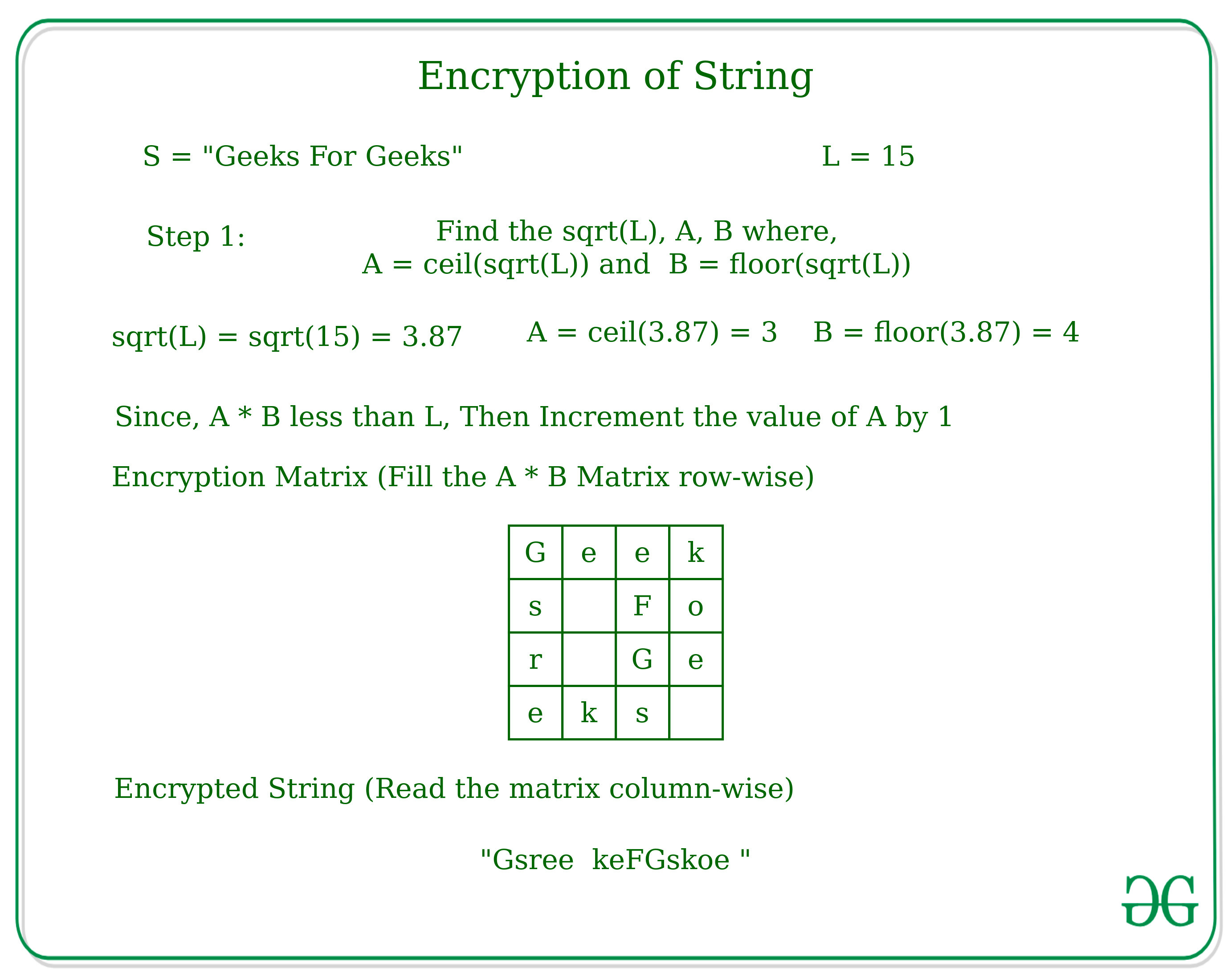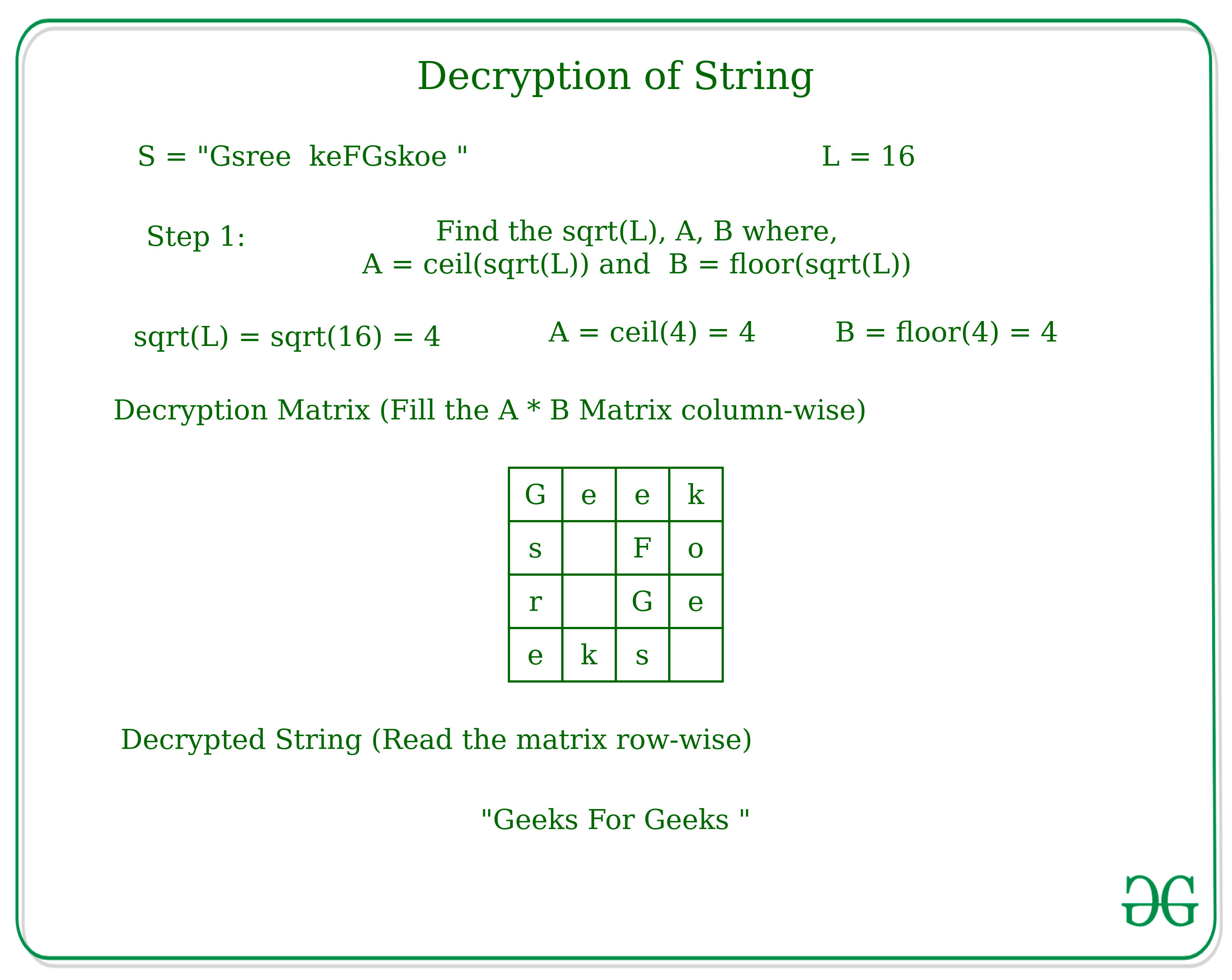Skip to content
Related Articles
Encryption and Decryption of String according to given technique
• Difficulty Level : Medium
• Last Updated : 31 May, 2021

Given a string S, the task is to encrypt the string and decrypt the string again to the original form.
Encryption Technique: If L is the length of the string, then take two values, one the ceil of √L (say b), and the other floor of √L (say a), and make a two-dimensional matrix having rows = a, and columns = b.
If rows*columns < L, then increase the value of a or b, whichever is minimum. Fill the matrix with the characters of the original string sequentially. After obtaining the matrix, read the matrix column-wise and print the obtained string.Decryption Technique: If L is the length of the encrypted string, then again find the two values a and b, where a is the ceil value of the √L and b is the floor value of the √L. Similarly create a 2D Matrix in which store the string column-wise and read the matrix row-wise to get the string in the original form.Encryption Approach:

• Find the length L of the string.
• Find the ceil and floor values of √Length and assign them to the variables.
• Check if the product of the two variables >= Length, if not then increments the variable having a lesser value by 1.
• Create a 2D matrix and fill the characters of the string row-wise.
• Read the matrix column-wise to get the encrypted string.

Decryption Approach:

• Find the length L of the string.
• Find the ceil and floor values of √Length and assign them to the variables.
• Create a 2D matrix and fill the matrix by characters of string column-wise.
• Read the matrix row-wise to get the decrypted string.

Below is the implementation of the above approach:

## C++

 `// C++ implementation for Custom``// Encryption and Decryption of String` `#include ``using` `namespace` `std;` `// Function to encrypt the string``string encryption(string s)``{``    ``int` `l = s.length();``    ``int` `b = ``ceil``(``sqrt``(l));``    ``int` `a = ``floor``(``sqrt``(l));``    ``string encrypted;``    ``if` `(b * a < l) {``        ``if` `(min(b, a) == b) {``            ``b = b + 1;``        ``}``        ``else` `{``            ``a = a + 1;``        ``}``    ``}` `    ``// Matrix to generate the``    ``// Encrypted String``    ``char` `arr[a][b];``    ``memset``(arr, ``' '``, ``sizeof``(arr));``    ``int` `k = 0;``    ` `    ``// Fill the matrix row-wise``    ``for` `(``int` `j = 0; j < a; j++) {``        ``for` `(``int` `i = 0; i < b; i++) {``            ``if` `(k < l){``                ``arr[j][i] = s[k];``            ``}``            ``k++;``        ``}``    ``}` `    ``// Loop to generate``    ``// encrypted string``    ``for` `(``int` `j = 0; j < b; j++) {``        ``for` `(``int` `i = 0; i < a; i++) {``            ``encrypted = encrypted +``                         ``arr[i][j];``        ``}``    ``}``    ``return` `encrypted;``}` `// Function to decrypt the string``string decryption(string s){``    ``int` `l = s.length();``    ``int` `b = ``ceil``(``sqrt``(l));``    ``int` `a = ``floor``(``sqrt``(l));``    ``string decrypted;` `    ``// Matrix to generate the``    ``// Encrypted String``    ``char` `arr[a][b];``    ``memset``(arr, ``' '``, ``sizeof``(arr));``    ``int` `k = 0;``    ` `    ``// Fill the matrix column-wise``    ``for` `(``int` `j = 0; j < b; j++) {``        ``for` `(``int` `i = 0; i < a; i++) {``            ``if` `(k < l){``                ``arr[j][i] = s[k];``            ``}``            ``k++;``        ``}``    ``}` `    ``// Loop to generate``    ``// decrypted string``    ``for` `(``int` `j = 0; j < a; j++) {``        ``for` `(``int` `i = 0; i < b; i++) {``            ``decrypted = decrypted +``                         ``arr[i][j];``        ``}``    ``}``    ``return` `decrypted;``}` `// Driver Code``int` `main()``{``    ``string s = ``"Geeks For Geeks"``;``    ``string encrypted;``    ``string decrypted;``    ` `    ``// Encryption of String``    ``encrypted = encryption(s);``    ``cout << encrypted << endl;``    ` `    ``// Decryption of String``    ``decrypted = decryption(encrypted);``    ``cout << decrypted;``    ``return` `0;``}`

## Java

 `// Java implementation for Custom``// Encryption and Decryption of String``class` `GFG``{` `// Function to encrypt the String``static` `String encryption(``char``[] s)``{``    ``int` `l = s.length;``    ``int` `b = (``int``) Math.ceil(Math.sqrt(l));``    ``int` `a = (``int``) Math.floor(Math.sqrt(l));``    ``String encrypted = ``""``;``    ``if` `(b * a < l)``    ``{``        ``if` `(Math.min(b, a) == b)``        ``{``            ``b = b + ``1``;``        ``}``        ``else``        ``{``            ``a = a + ``1``;``        ``}``    ``}` `    ``// Matrix to generate the``    ``// Encrypted String``    ``char` `[][]arr = ``new` `char``[a][b];``    ``int` `k = ``0``;``    ` `    ``// Fill the matrix row-wise``    ``for` `(``int` `j = ``0``; j < a; j++)``    ``{``        ``for` `(``int` `i = ``0``; i < b; i++)``        ``{``            ``if` `(k < l)``            ``{``                ``arr[j][i] = s[k];``            ``}``            ``k++;``        ``}``    ``}` `    ``// Loop to generate``    ``// encrypted String``    ``for` `(``int` `j = ``0``; j < b; j++)``    ``{``        ``for` `(``int` `i = ``0``; i < a; i++)``        ``{``            ``encrypted = encrypted +``                        ``arr[i][j];``        ``}``    ``}``    ``return` `encrypted;``}` `// Function to decrypt the String``static` `String decryption(``char` `[]s)``{``    ``int` `l = s.length;``    ``int` `b = (``int``) Math.ceil(Math.sqrt(l));``    ``int` `a = (``int``) Math.floor(Math.sqrt(l));``    ``String decrypted=``""``;` `    ``// Matrix to generate the``    ``// Encrypted String``    ``char` `[][]arr = ``new` `char``[a][b];``    ``int` `k = ``0``;``    ` `    ``// Fill the matrix column-wise``    ``for` `(``int` `j = ``0``; j < b; j++)``    ``{``        ``for` `(``int` `i = ``0``; i < a; i++)``        ``{``            ``if` `(k < l)``            ``{``                ``arr[j][i] = s[k];``            ``}``            ``k++;``        ``}``    ``}` `    ``// Loop to generate``    ``// decrypted String``    ``for` `(``int` `j = ``0``; j < a; j++)``    ``{``        ``for` `(``int` `i = ``0``; i < b; i++)``        ``{``            ``decrypted = decrypted +``                        ``arr[i][j];``        ``}``    ``}``    ``return` `decrypted;``}` `// Driver Code``public` `static` `void` `main(String[] args)``{``    ``String s = ``"Geeks For Geeks"``;``    ``String encrypted;``    ``String decrypted;``    ` `    ``// Encryption of String``    ``encrypted = encryption(s.toCharArray());``    ``System.out.print(encrypted +``"\n"``);``    ` `    ``// Decryption of String``    ``decrypted = decryption(encrypted.toCharArray());``    ``System.out.print(decrypted);``}``}` `// This code is contributed by PrinciRaj1992`

## Python3

 `# Python3 implementation for Custom``# Encryption and Decryption of String``from` `math ``import` `ceil,floor,sqrt` `# Function to encrypt the``def` `encryption(s):``    ``l ``=` `len``(s)``    ``b ``=` `ceil(sqrt(l))``    ``a ``=` `floor(sqrt(l))``    ``encrypted``=``""``    ``if` `(b ``*` `a < l):``        ``if` `(``min``(b, a) ``=``=` `b):``            ``b ``=` `b ``+` `1``        ``else``:``            ``a ``=` `a ``+` `1` `    ``# Matrix to generate the``    ``# Encrypted String``    ``arr ``=` `[[``' '` `for` `i ``in` `range``(a)] ``for` `j ``in` `range``(b)]``    ``k ``=` `0` `    ``# Fill the matrix row-wise``    ``for` `j ``in` `range``(a):``        ``for` `i ``in` `range``(b):``            ``if` `(k < l):``                ``arr[j][i] ``=` `s[k]``            ``k ``+``=` `1` `    ``# Loop to generate``    ``# encrypted``    ``for` `j ``in` `range``(b):``        ``for` `i ``in` `range``(a):``            ``encrypted ``=` `encrypted ``+` `arr[i][j]` `    ``return` `encrypted` `# Function to decrypt the``def` `decryption(s):``    ``l ``=` `len``(s)``    ``b ``=` `ceil(sqrt(l))``    ``a ``=` `floor(sqrt(l))``    ``decrypted``=``""` `    ``# Matrix to generate the``    ``# Encrypted String``    ``arr ``=` `[[``' '` `for` `i ``in` `range``(a)] ``for` `j ``in` `range``(b)]``    ``k ``=` `0` `    ``# Fill the matrix column-wise``    ``for` `j ``in` `range``(b):``        ``for` `i ``in` `range``(a):``            ``if` `(k < l):``                ``arr[j][i] ``=` `s[k]``            ``k ``+``=` `1` `    ``# Loop to generate``    ``# decrypted``    ``for` `j ``in` `range``(a):``        ``for` `i ``in` `range``(b):``            ``decrypted ``=` `decrypted ``+` `arr[i][j]``    ``return` `decrypted` `# Driver Code` `s ``=` `"Geeks For Geeks"``encrypted``=``""``decrypted``=``""` `# Encryption of String``encrypted ``=` `encryption(s)``print``(encrypted)` `# Decryption of String``decrypted ``=` `decryption(encrypted)``print``(decrypted)` `# This code is contributed by mohit kumar 29`

## C#

 `// C# implementation for Custom``// Encryption and Decryption of String``using` `System;` `class` `GFG``{`` ` `// Function to encrypt the String``static` `String encryption(``char``[] s)``{``    ``int` `l = s.Length;``    ``int` `b = (``int``) Math.Ceiling(Math.Sqrt(l));``    ``int` `a = (``int``) Math.Floor(Math.Sqrt(l));``    ``String encrypted = ``""``;``    ``if` `(b * a < l)``    ``{``        ``if` `(Math.Min(b, a) == b)``        ``{``            ``b = b + 1;``        ``}``        ``else``        ``{``            ``a = a + 1;``        ``}``    ``}`` ` `    ``// Matrix to generate the``    ``// Encrypted String``    ``char` `[,]arr = ``new` `char``[a, b];``    ``int` `k = 0;``     ` `    ``// Fill the matrix row-wise``    ``for` `(``int` `j = 0; j < a; j++)``    ``{``        ``for` `(``int` `i = 0; i < b; i++)``        ``{``            ``if` `(k < l)``            ``{``                ``arr[j, i] = s[k];``            ``}``            ``k++;``        ``}``    ``}`` ` `    ``// Loop to generate``    ``// encrypted String``    ``for` `(``int` `j = 0; j < b; j++)``    ``{``        ``for` `(``int` `i = 0; i < a; i++)``        ``{``            ``encrypted = encrypted +``                        ``arr[i, j];``        ``}``    ``}``    ``return` `encrypted;``}`` ` `// Function to decrypt the String``static` `String decryption(``char` `[]s)``{``    ``int` `l = s.Length;``    ``int` `b = (``int``) Math.Ceiling(Math.Sqrt(l));``    ``int` `a = (``int``) Math.Floor(Math.Sqrt(l));``    ``String decrypted=``""``;`` ` `    ``// Matrix to generate the``    ``// Encrypted String``    ``char` `[,]arr = ``new` `char``[a, b];``    ``int` `k = 0;``     ` `    ``// Fill the matrix column-wise``    ``for` `(``int` `j = 0; j < b; j++)``    ``{``        ``for` `(``int` `i = 0; i < a; i++)``        ``{``            ``if` `(k < l)``            ``{``                ``arr[j, i] = s[k];``            ``}``            ``k++;``        ``}``    ``}`` ` `    ``// Loop to generate``    ``// decrypted String``    ``for` `(``int` `j = 0; j < a; j++)``    ``{``        ``for` `(``int` `i = 0; i < b; i++)``        ``{``            ``decrypted = decrypted +``                        ``arr[i, j];``        ``}``    ``}``    ``return` `decrypted;``}`` ` `// Driver Code``public` `static` `void` `Main(String[] args)``{``    ``String s = ``"Geeks For Geeks"``;``    ``String encrypted;``    ``String decrypted;``     ` `    ``// Encryption of String``    ``encrypted = encryption(s.ToCharArray());``    ``Console.Write(encrypted +``"\n"``);``     ` `    ``// Decryption of String``    ``decrypted = decryption(encrypted.ToCharArray());``    ``Console.Write(decrypted);``}``}` `// This code is contributed by PrinciRaj1992`

## Javascript

 ``
Output:
```Gsree  keFGskoe
Geeks For Geeks```

Attention reader! Don’t stop learning now. Get hold of all the important DSA concepts with the DSA Self Paced Course at a student-friendly price and become industry ready.  To complete your preparation from learning a language to DS Algo and many more,  please refer Complete Interview Preparation Course.

In case you wish to attend live classes with industry experts, please refer DSA Live Classes

My Personal Notes arrow_drop_up